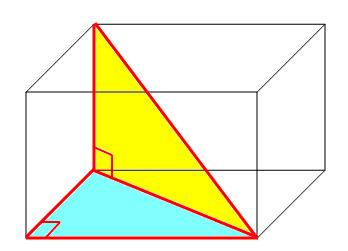# XYZ Plane

## Homework Statement

A room has dimensions 2.85 m (height) × 5.93 m × 6.29 m. A fly starting at one corner flies around, ending up at the diagonally opposite corner. (a) What is the magnitude of its displacement? (b) If the fly walks rather than flies, what is the length of the shortest path it can take? (Hint: This can be answered without calculus. The room is like a box. Unfold its walls to flatten them into a plane.)

## Homework Equations

HINT:Put the origin of an xyz coordinate system at the starting corner; let the axes extend along the room edges.

To find the shortest path for walking, unfold the room like a box, laying out its sides and marking the start and finish points. If you unfold it the right way, you need only the Pythagorean theorem.

## The Attempt at a Solution

For Part A, I got 6.58 m, but this answer came up wrong on the HW program.

Redbelly98
Staff Emeritus
Homework Helper
Can you show your work? Then we try to see where things went wrong.

I set up the XYZ plane. 2.85 was the Z axis..and 5.93 and the 6.29 were the X and Y axis. I basically made a triangle from one corner to the other. I did 2.85^2+5.93^2=magnitude of one displacement. That was 6.58. That is pretty much as far as I have gotten on this problem. I don't know if i set up another triangle or not. I just can't visualize the path the fly took. It's hard to put on paper.

Redbelly98
Staff Emeritus
Homework Helper
I found this image, which may be helpful:You have found the hypotenuse of the blue triangle in the figure (6.58 m). You can use that information to find the desired distance, which is the hypotenuse of the yellow triangle here.

Yes! I got 9.10, which was the correct answer. Now how do I come across part B?

Redbelly98
Staff Emeritus